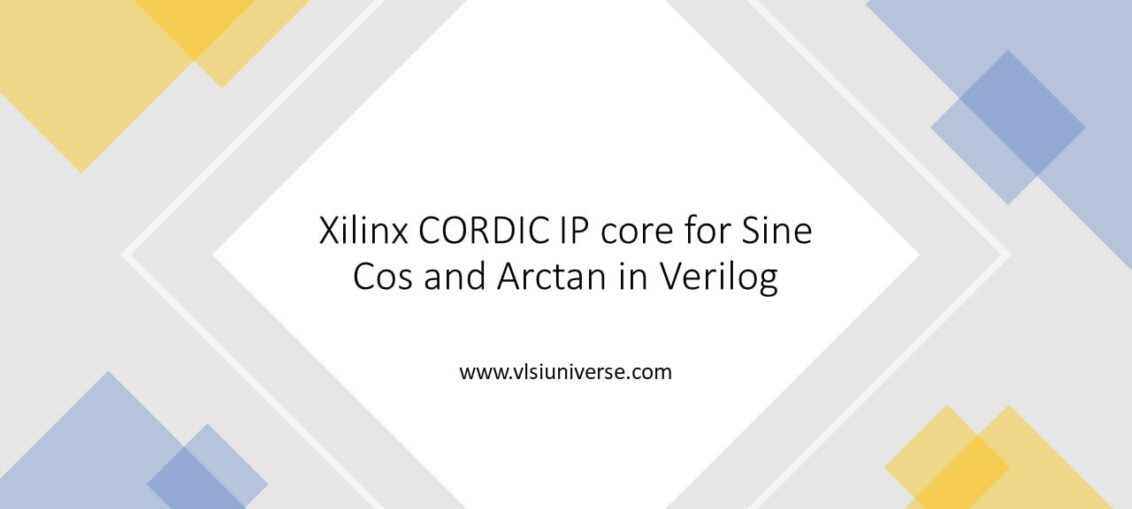You are here
Home > Verilog >

# Verilog code for sine cos and arctan using CORDIC AlgorithmHardware implementation of trigonometric functions such as sine, cosine and tangent functions using Verilog HDL. Here we make use of CORDIC algorithm to implement these functions in Verilog. It is important to to write a synthesizable code in Verilog to implement on hardware such as FPGA’s.

The computation of the functions helps to analyze sine wave generation and phase and magnitude calculation of several signals.

## 1. Basics of CORDIC Algorithm

### 1.1 Need for CORDIC Algorithm

There are several approaches such as the Lookup table method and Polynomial series (for example, Taylor series) to implement these sine, cosine and tangent trigonometric functions. They have complications such as cost of the implementation and the hardware complexity of the design.

In case Lookup table method it is fast but as the precision of the input angle increases the table size increases exponentially. And In case of Taylor’s series method it is slow and require more hardware to implement. Hence there a need for an low cost and faster algorithm to implement these functions.

### 1.2 Benefits of using CORDIC algorithm

It is a adder/subtractor and shift register based algorithm which does not need multipliers or dividers which makes the task easy. The name CORDIC come form Co-ordinate Rotational Digital Computer.

It also require a small lookup table along with adders and shifters which makes it very hardware efficient and is faster can be used for FPGA Implementation.

## 2. Working of CORDIC Algorithm

The algorithm is mainly based on rotating a point (x1,y1) to point (x2,y2) with an angle of difference “Ɵ“, as shown in the below figure.X2 = X1cosθ – Y1sinθ
Y2 = X1sinθ + Y1cosθ  ——————- (1)

The key concept of the CORDIC algorithm is to rotate the point (X1,Y1) by standard angles of θ, where tanθ = 2^(−𝑖). Therefore the above equations change to,

X2 = X1 – Y1tanθ
Y2 = X1 + Y1tanθ ————————–(2)

The CORDIC algorithm can be made to work with two modes of operation. Rotating mode of CORDIC algorithm helps to evaluate trigonometric functions (cosine and sine).

Where as Vectoring mode of CORDIC algorithm helps to evaluate magnitude and phase of a complex signal by computing tangent function.

## 2.1 Rotating mode of CORDIC Algorithm

CORDIC algorithm is a iterative rotation algorithm, where successive rotations are made to a vector point (X,Y) with an angles (standard tanθ = 2^(−𝑖) where i = 0,1,2,3..) until the desired angle is reached.

Here each successive step calculates a rotation by multiplying the previous vector Vi-1 with some scale Ri (Rotation matrix). The next vector Vi is given by,

Vi=Vi-1 x Ri

The scaling rotation matrix Ri is given as below,

Further to have the rotation matrix in the for of tangent functions, the above equation can be modified asIn the above equation the values Xi-1 and Yi-1 are the factors of tangent function tanθ = 2^(−𝑖). Replacing the tan function with 2^(-i)^2 which is nothing but 2^(-2i) in the scale factor. The modified scale factor Ki will be as below,In the above equation, the value of scale factor Ki reaches 0.6073 approximately, when the i value goes to infinity. Means infinite number of iterations.
Replacing the Ki with the value 0.6073, the gain becomes 1.647.## 2.2 Vectoring mode of CORDIC Algorithm

With the slight modification in the CORDIC algorithm, the CORDIC algorithm can be mode to work in the vectoring mode.
The Vector with X is in the positive co-ordinate and have the Y axis as arbitrary. The main goal is to have as many as rotations of X axis until the Y coordinate reaches to zero.

At each iteration the Y coordinate value will determine the direction in which rotation has to be carried out.
The final βi value will be the desired total angle of rotation.
The final x value will be the magnitude of the vector multiplied by K i.e. K(x^2+y^2).
The below table summarizes the rotation mode and vectoring mode of CORDIC algorithm.

### The function arctan implementation in CORDIC algorithm

The trigonometric function arccos (cos inverse) implementation using arctan (tan inverse) by vectoring mode of CORDIC algorithm in Verilog.

arccos(x) = arctan(√(𝟏−𝒙^𝟐 )/𝒙) (1)

Input angle is given in the form of x*1000 where x represents input in radian further this gain is compensated in the equation (√(1−𝑥^2 )/𝑥).

The phase output is scaled with a gain of 1000.

Let us now write a complete and synthesizable Verilog HDL code for all three trigonometric functions.

## 3. Sine and Cosine Implementation in Verilog using CORDIC algorithm

1. Cosine & Sine implementation using rotating mode of CORDIC in Verilog.
2. Input angle is given in the form of (2^32*i)/360 where i represents input phase angle in degree.
3. The output is scaled with a gain of 32000.### Sine and Cosine Output Simulation Waveform### Below is the Table for comparison of results with theoretical values## 4. arctan implementation in Verilog using CORDIC algorithm

1. Arccos(cos inverse) implementation using arctan(tan inverse) by vectoring mode of CORDIC algorithm in Verilog.
arccos(x) = arctan(√(1−𝑥^2 )/𝑥) ………………………….(1)
2. Input angle is given in the form of x*1000 where x represents input in radian further this gain is compensated in the equation (√(1−𝑥^2 )/𝑥).
3. The phase output is scaled with a gain of 342068275.